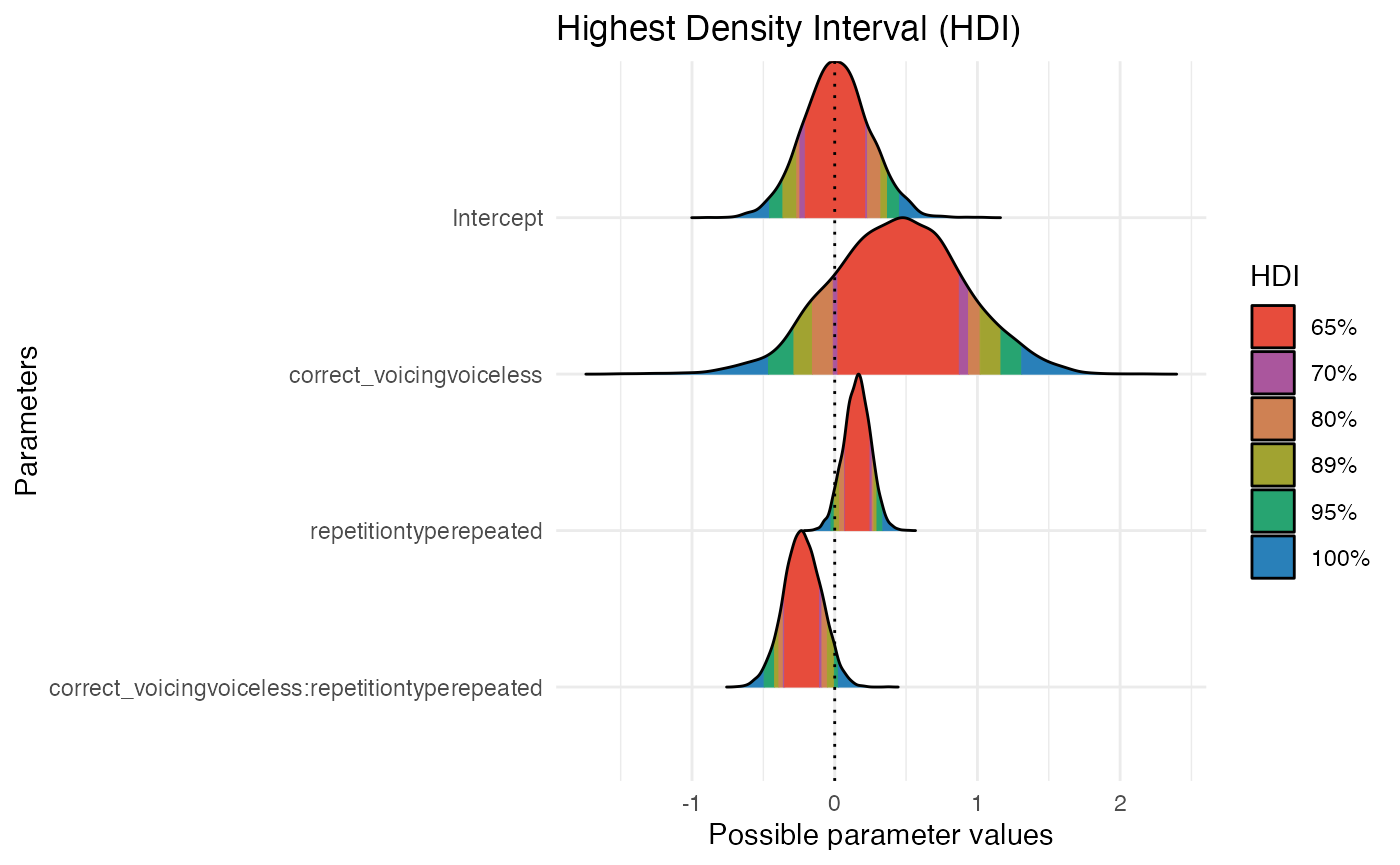library(tidyverse)
theme_set(theme_minimal())
library(ggrepel)
library(learnB4SS)
library(HDInterval)
library(tidybayes)
library(bayesplot)
library(modelr)
library(broom.mixed)
library(brms)

data("incomplete")

# Set custom b4ss colours
b4ss_colors <- c(purple = "#8970FF", orange = "#FFA70B")
scale_fill_b4ss <- function(...) {
scale_fill_manual(..., values = c(b4ss_colors[], b4ss_colors[]))
}
scale_color_b4ss <- function(...) {
scale_color_manual(..., values = c(b4ss_colors[], b4ss_colors[]))
}

# Study overview and data

glimpse(incomplete)
#> Rows: 6,144
#> Columns: 8
#> $order <dbl> 9, 10, 11, 12, 13, 14, 15, 16, 17, 18, 19, 20, 21, 22,… #>$ speaker_voice   <chr> "VP11", "VP10", "VP07", "VP15", "VP06", "VP09", "VP08"…
#> $item_pair <dbl> 19, 11, 14, 23, 7, 15, 3, 13, 24, 2, 5, 20, 3, 8, 4, 9… #>$ RT              <dbl> 1531, 1009, 633, 1852, 3606, 4493, 15186, 1995, 940, 4…
#> $correct <dbl> 1, 1, 1, 1, 0, 1, 0, 0, 0, 0, 0, 0, 1, 1, 0, 1, 1, 1, … #>$ correct_voicing <chr> "voiceless", "voiceless", "voiced", "voiced", "voicele…
#> $listener <chr> "L01", "L01", "L01", "L01", "L01", "L01", "L01", "L01"… #>$ repetitiontype  <chr> "first", "first", "first", "first", "first", "first", …

# Model formula

m1_bf <- brmsformula(
correct ~
correct_voicing *
repetitiontype +
# random slopes for interaction across listeners
(correct_voicing * repetitiontype | listener) +
# random slopes for interaction across speaker voices
(correct_voicing * repetitiontype | speaker_voice) +
# random slopes for interaction across minimal pairs
(correct_voicing * repetitiontype | item_pair),
family = bernoulli()
)

# Priors and prior predictive checks

## Prior distribution of the outcome variable (likelihood, family)

$correct_i \sim Bernoulli(p)$

y <- dbern(c(0, 1), p = 0.75)

ggplot() +
aes(c("0", "1"), y) +
geom_linerange(aes(ymin = 0, ymax = y), size = 2, colour = b4ss_colors) +
geom_point(size = 5, colour = b4ss_colors) +
ylim(0, 1) +
labs(x = "outcome", y = "p")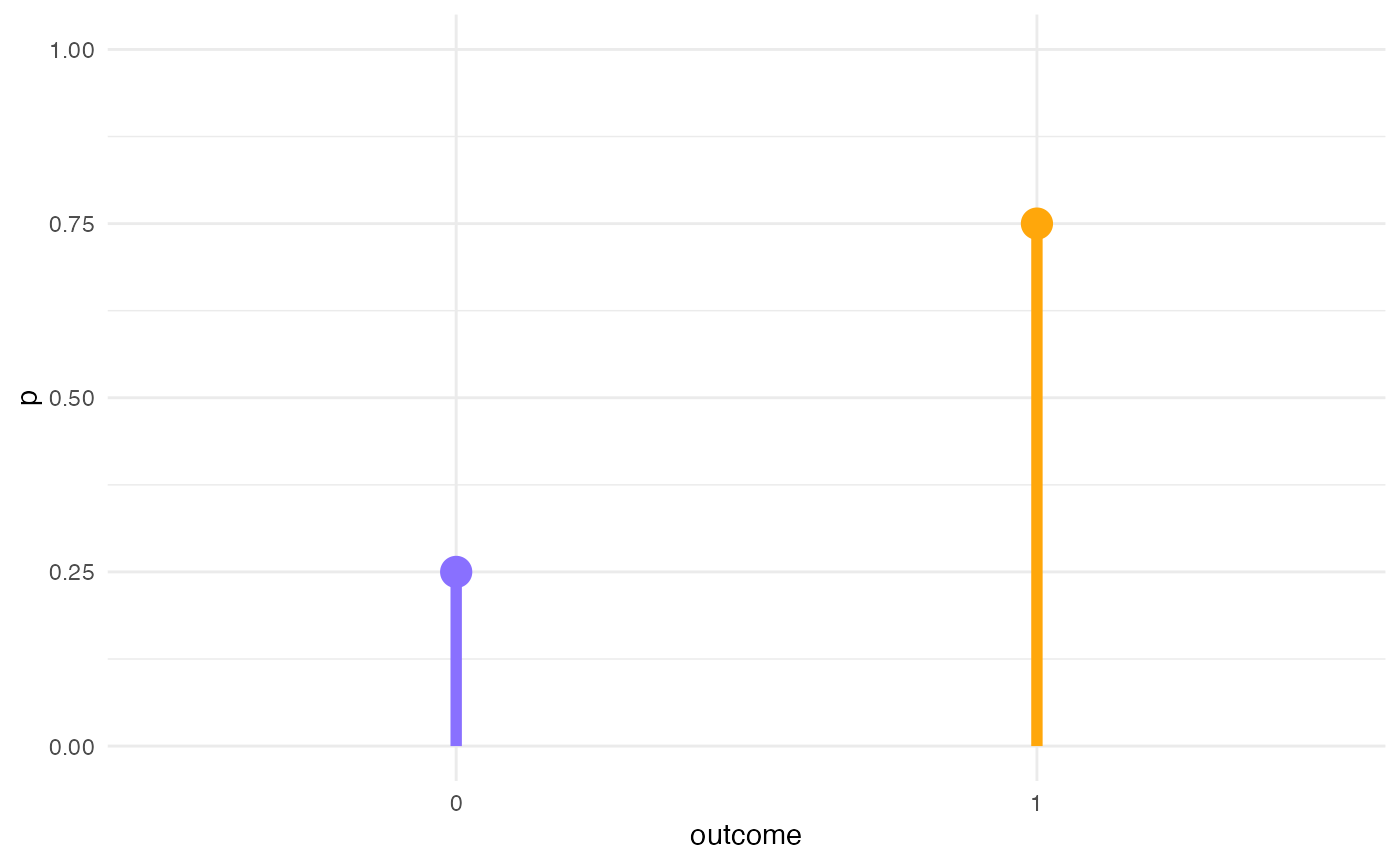## Get priors

# get_prior(m1_bf, data = incomplete)
get_prior(m1_bf, data = incomplete) %>%
as_tibble() %>%
select(prior:group)
#> # A tibble: 25 x 4
#>    prior              class    coef                                 group
#>    <chr>              <chr>    <chr>                                <chr>
#>  1 ""                 b        ""                                   ""
#>  2 ""                 b        "correct_voicingvoiceless"           ""
#>  3 ""                 b        "correct_voicingvoiceless:repetitio… ""
#>  4 ""                 b        "repetitiontyperepeated"             ""
#>  5 "lkj(1)"           cor      ""                                   ""
#>  6 ""                 cor      ""                                   "item_pair"
#>  7 ""                 cor      ""                                   "listener"
#>  8 ""                 cor      ""                                   "speaker_vo…
#>  9 "student_t(3, 0, … Interce… ""                                   ""
#> 10 "student_t(3, 0, … sd       ""                                   ""
#> # … with 15 more rows

## Log-odds space

dots <- tibble(
p = seq(0.1, 0.9, by = 0.1),
log_odds = qlogis(p)
)

tibble(
p = seq(0, 1, by = 0.001),
log_odds = qlogis(p)
) %>%
ggplot(aes(p, log_odds)) +
geom_hline(yintercept = 0, alpha = 0.5) +
geom_vline(xintercept = 0.5, linetype = "dashed") +
geom_line(size = 2, colour = b4ss_colors) +
geom_point(data = dots, size = 4, colour = b4ss_colors) +
scale_x_continuous(breaks = seq(0, 1, by = 0.1), minor_breaks = NULL) +
scale_y_continuous(breaks = seq(-6, 6, by = 2), minor_breaks = NULL) +
labs(
title = "Correspondence between probabilities and log-odds",
x = "probability",
y = "log-odds"
)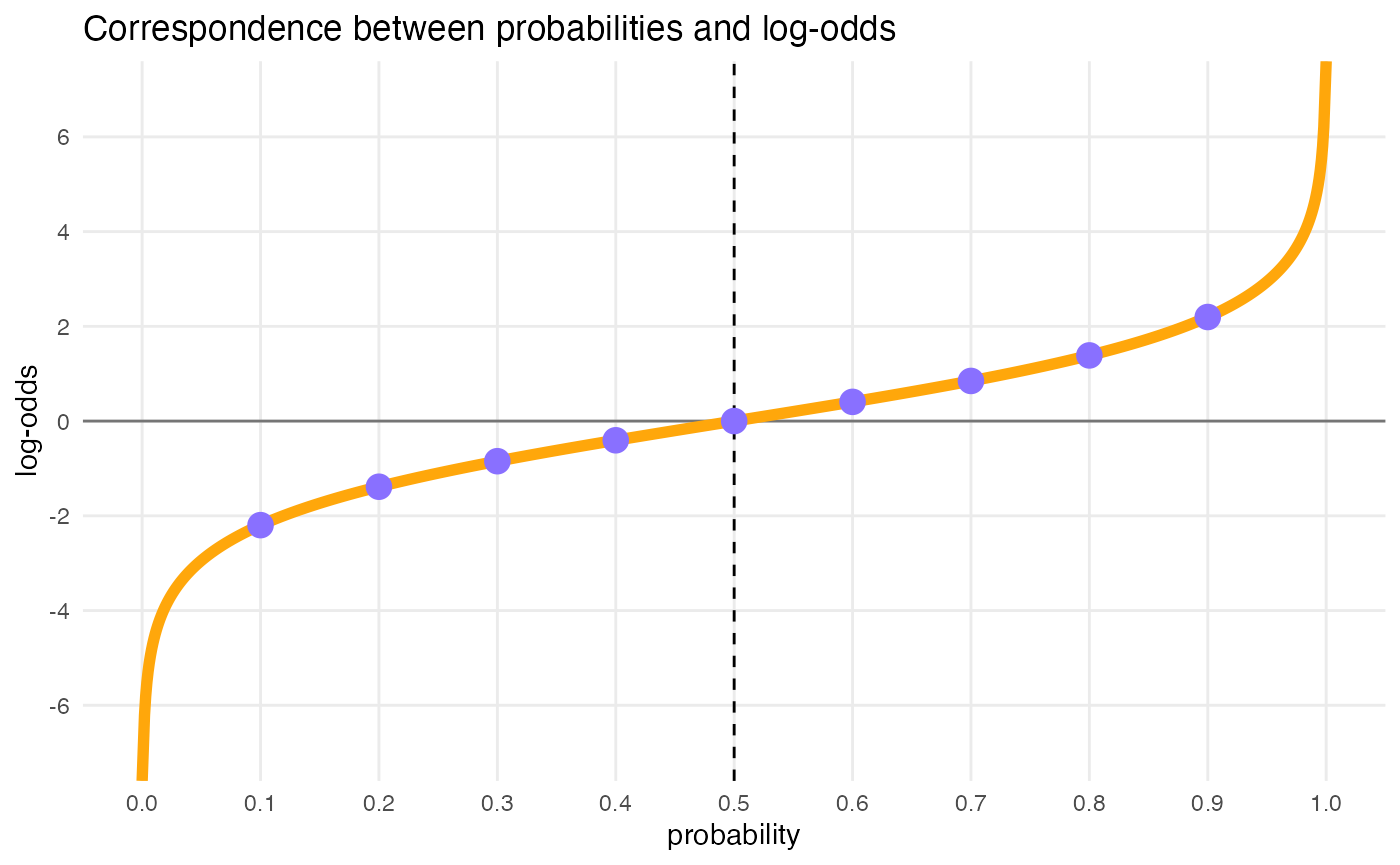# log-odds = 0; get probability
glue::glue("Log-odds 0 = p(", plogis(0), ")")
#> Log-odds 0 = p(0.5)
# p = 0.5; get log-odds
glue::glue("p(0.5) = log-odds ", qlogis(0.5))
#> p(0.5) = log-odds 0

cat("\n\n")

# log-odds = 1.5; get probability
glue::glue("Log-odds 1.5 = p(", round(plogis(1.5), 4), ")")
#> Log-odds 1.5 = p(0.8176)
# p = 0.5; get log-odds
glue::glue("p(0.8176) = log-odds ", round(qlogis(0.8175745), 4))
#> p(0.8176) = log-odds 1.5

## Prior for the intercept

# Log-odds to p
plogis(-6) # ~= 0
#>  0.002472623
plogis(6) # ~= 1
#>  0.9975274
x <- seq(-15, 15, by = 0.1)
# 95% Cri [-6, +6] => SD = 6/2 = 3
y <- dnorm(x, mean = 0, sd = 3)

ggplot() +
aes(x, y) +
geom_line(size = 1.5, colour = b4ss_colors) +
labs(x = "log-odds")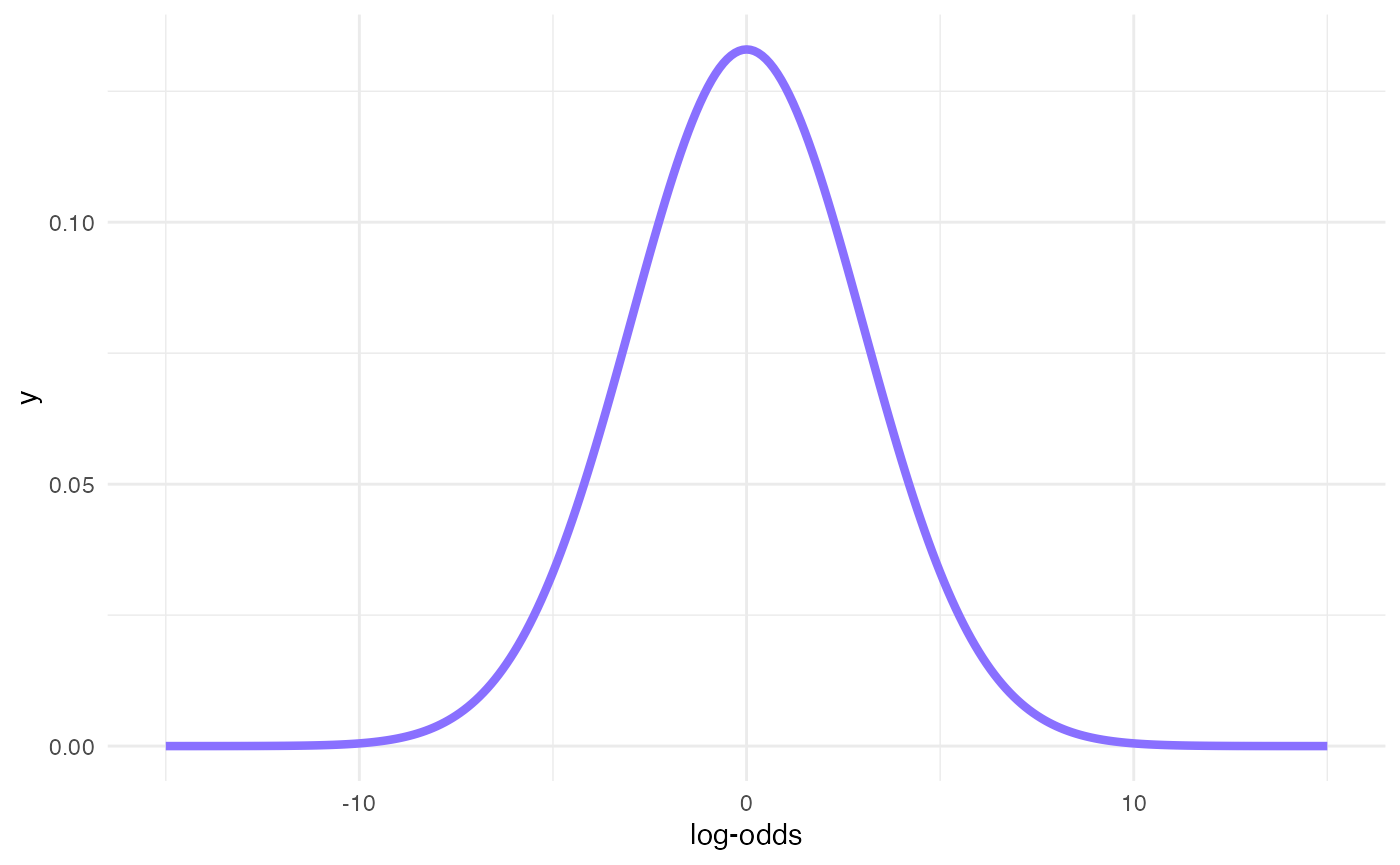## Prior for b

# Log-odds to odds
exp(-2) # ~= 0
#>  0.1353353
exp(2) # ~= 7
#>  7.389056
x <- seq(-4, 4, by = 0.1)
# 95% Cri [-2, +2] => SD = 2/2 = 1
y <- dnorm(x, mean = 0, sd = 1)

ggplot() +
aes(x, y) +
geom_line(size = 1.5, colour = b4ss_colors) +
labs(x = "log-odds")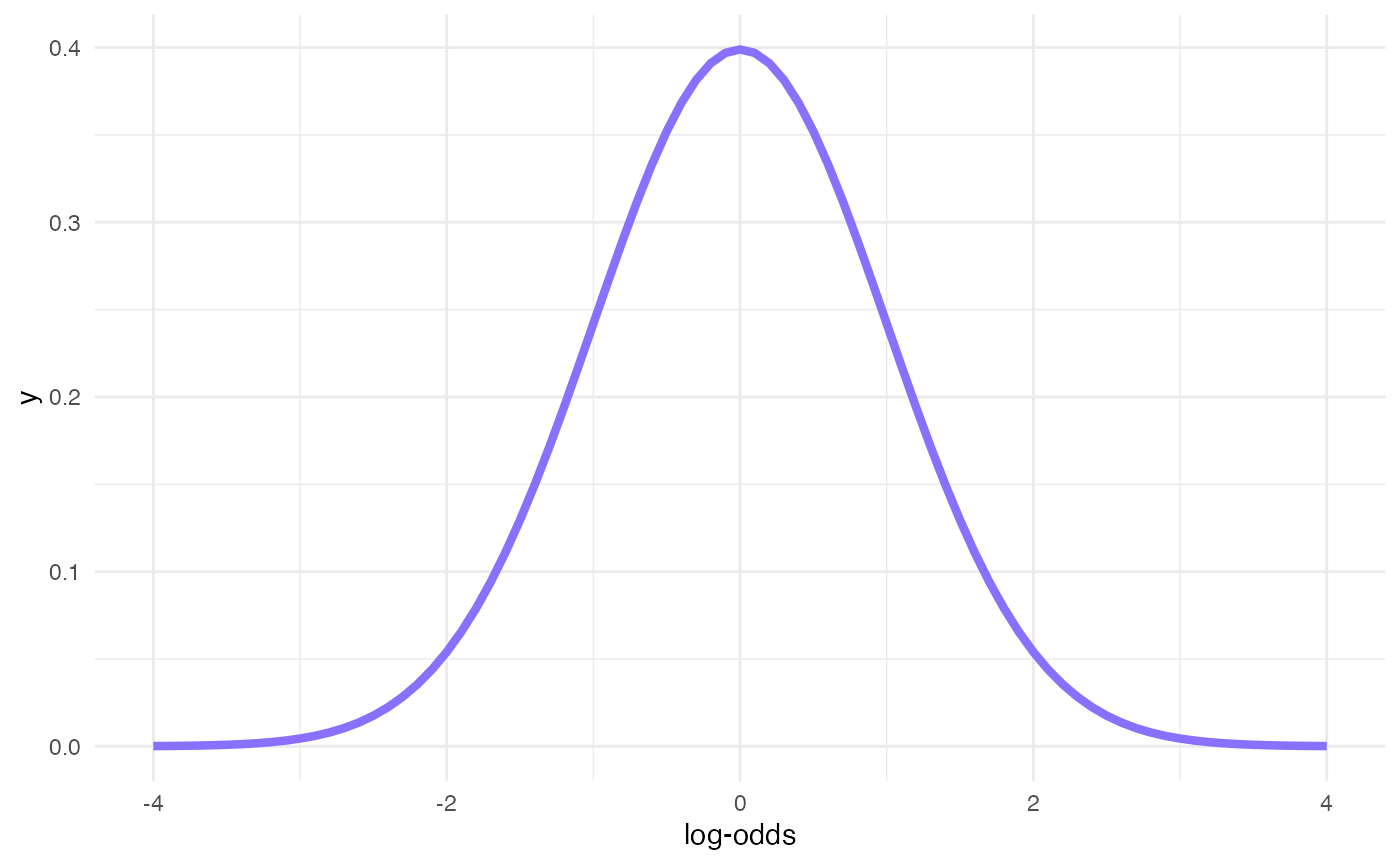## Prior for sd

inverseCDF(c(0.025, 0.975), phcauchy, sigma = 0.1)
#>  0.003930135 2.545175934
x <- seq(0, 3, by = 0.01)
y <- dhcauchy(x, sigma = 0.1)

ggplot() +
aes(x, y) +
geom_line(size = 1, colour = b4ss_colors) +
labs(x = "log-odds")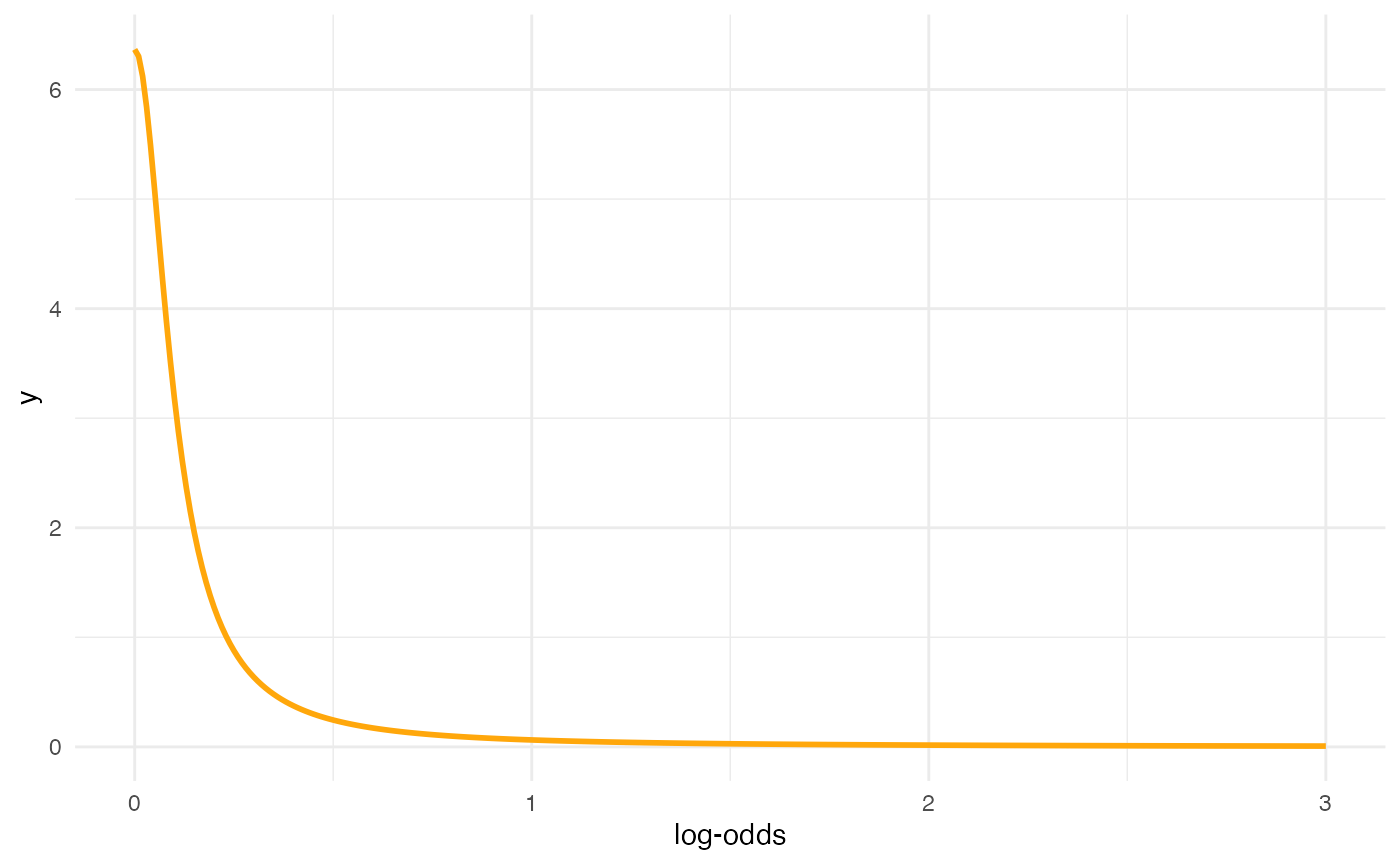## Set priors

priors <- c(
prior(normal(0, 3), class = Intercept),
prior(normal(0, 1), class = b),
prior(cauchy(0, 0.1), class = sd),
prior(lkj(2), class = cor)
)

## Prior predictive checks

m1_priorpc <- brm(
m1_bf,
data = incomplete,
prior = priors,
sample_prior = "only",
file = system.file("extdata/m1_priorpc.rds", package = "learnB4SS")
)
conditional_effects(m1_priorpc, effects = "correct_voicing:repetitiontype")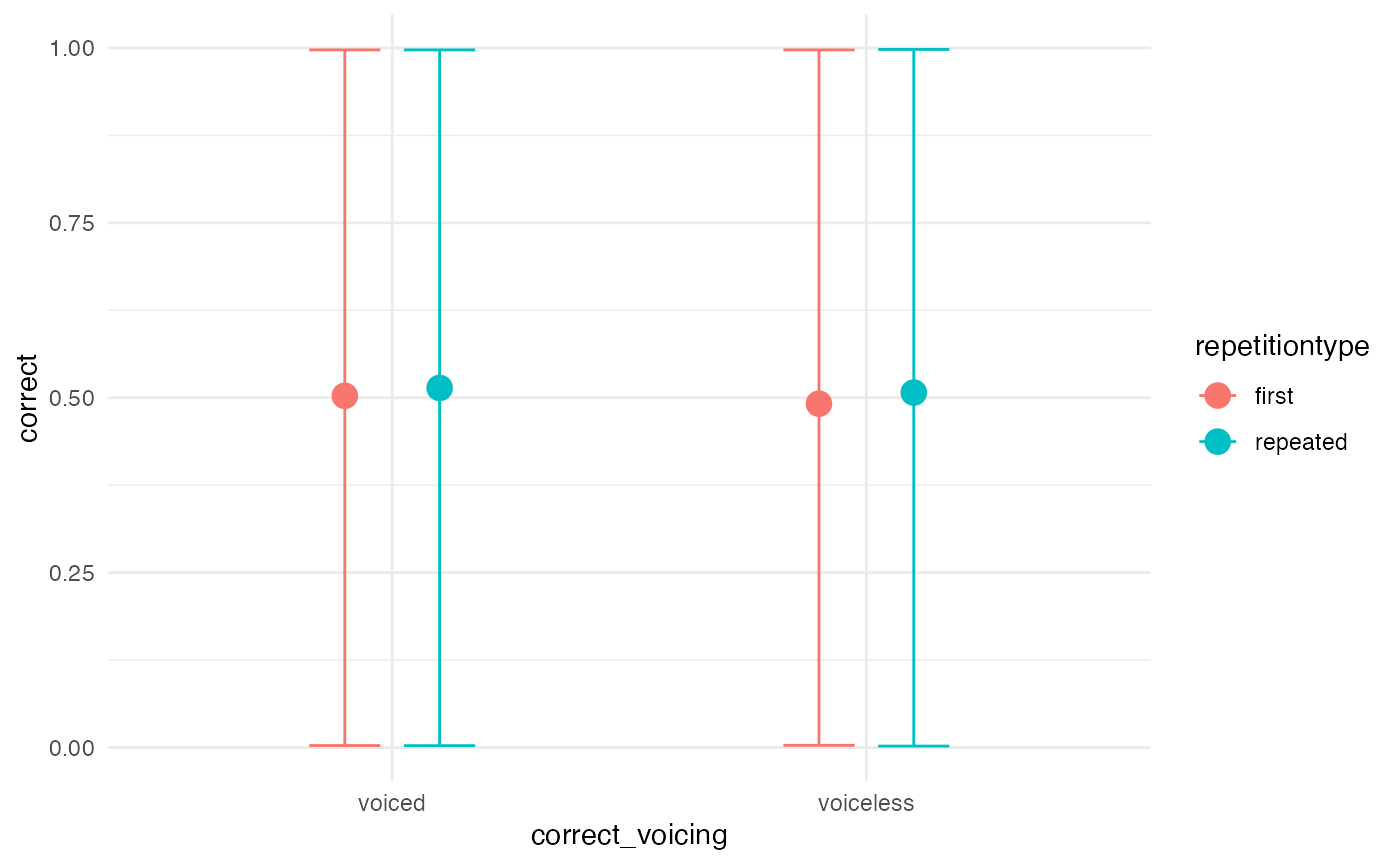## Let’s try VERY strong priors for comparison

priors_strong <- c(
prior(normal(2, 0.1), class = Intercept),
prior(normal(1, 0.1), class = b),
prior(cauchy(0, 0.1), class = sd),
prior(lkj(2), class = cor)
)
m1_priorpc_strong <- brm(
m1_bf,
data = incomplete,
prior = priors_strong,
sample_prior = "only",
cores = 4,
file = system.file("extdata/m1_priorpc_strong.rds", package = "learnB4SS")
)
conditional_effects(m1_priorpc_strong, effects = "correct_voicing:repetitiontype")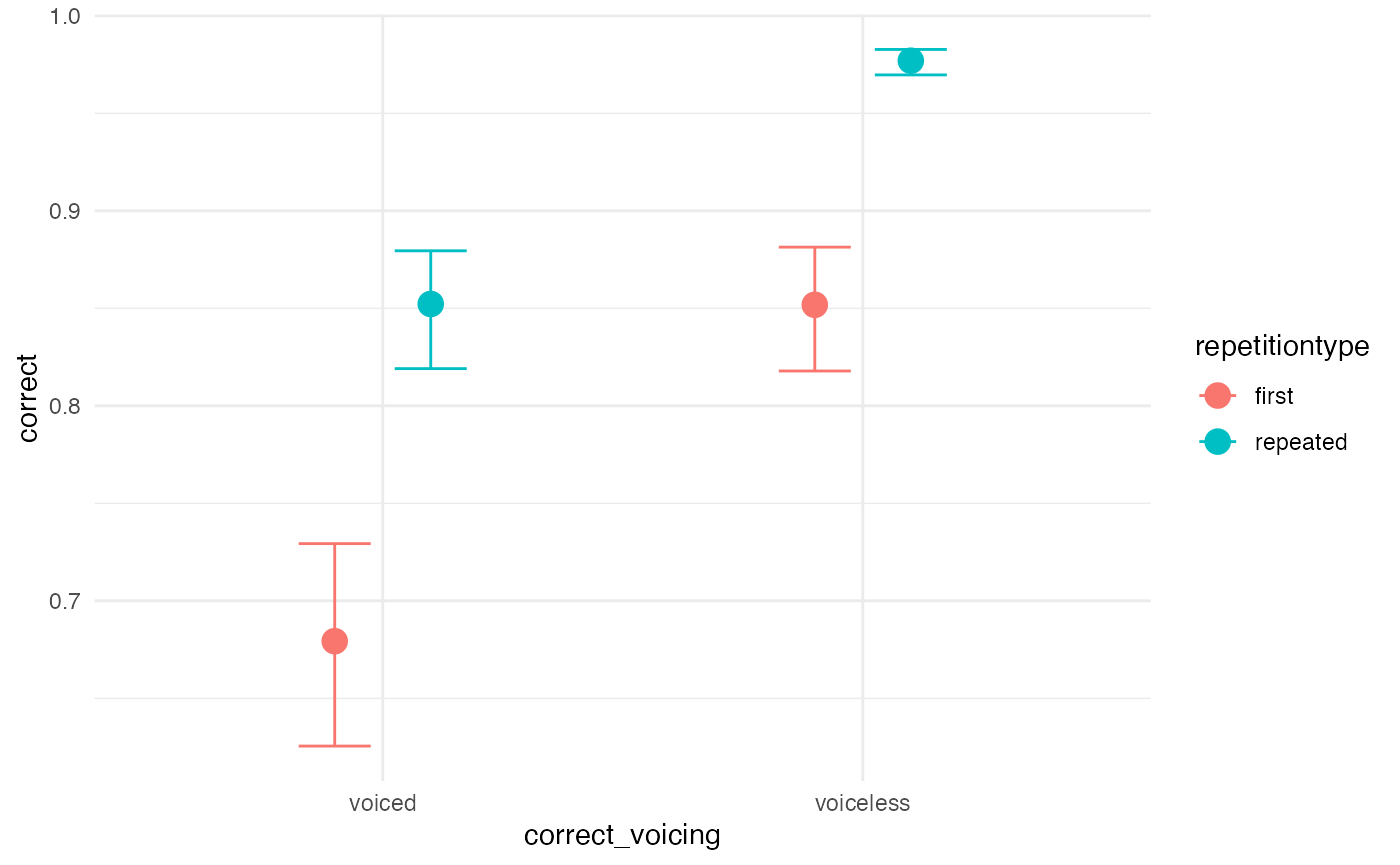# Run model

m1_full <- brm(
m1_bf,
data = incomplete,
prior = priors,
cores = parallel::detectCores(),
chains = 4,
iter = 2000,
warmup = 1000,
file = system.file("extdata/m1_full.rds", package = "learnB4SS")
)

# Model output and interpretation

## Checks and diagnostics

# Sample data from the generative model and compare with real data
pp_check(m1_full, nsamples = 200)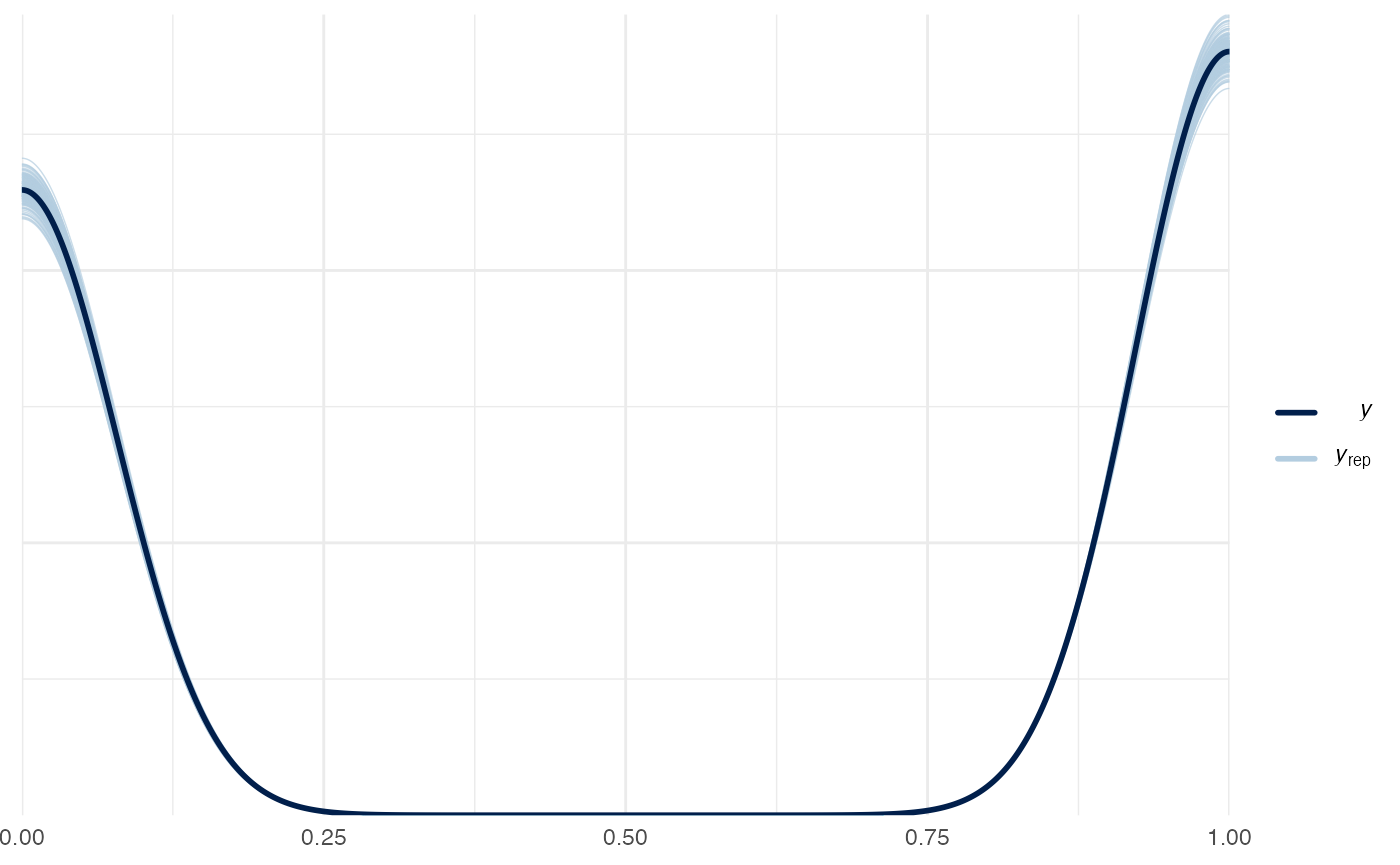# Generate trace and density plots for MCMC samples
# Only looking at pop-level parameters (no SDs or cor)
plot(m1_full, pars = "^b_")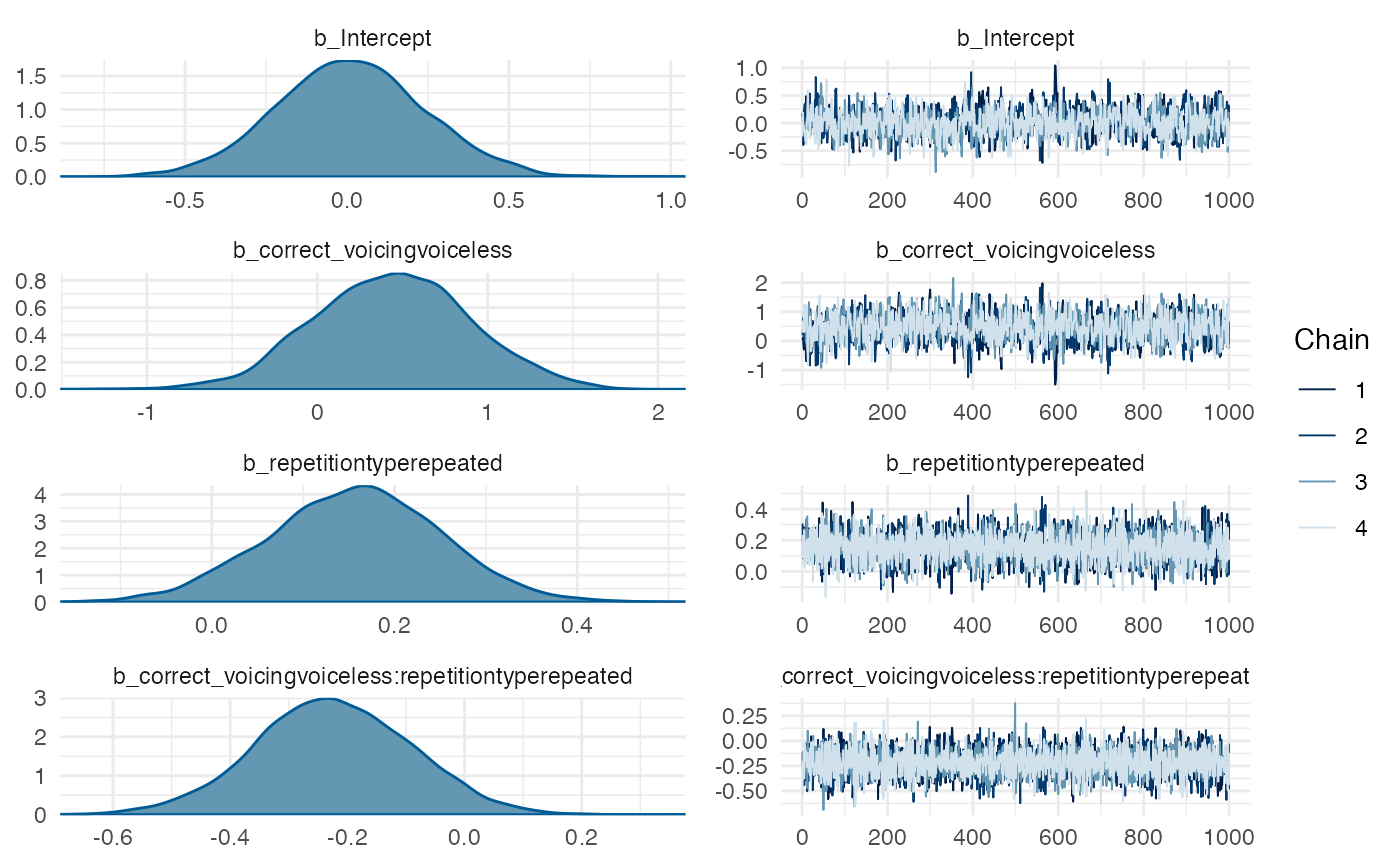# Pairs plots to help spot degeneracies (doesn't apply here but
# useful if you have divergent transitions)
pairs(m1_full, pars = "^b_")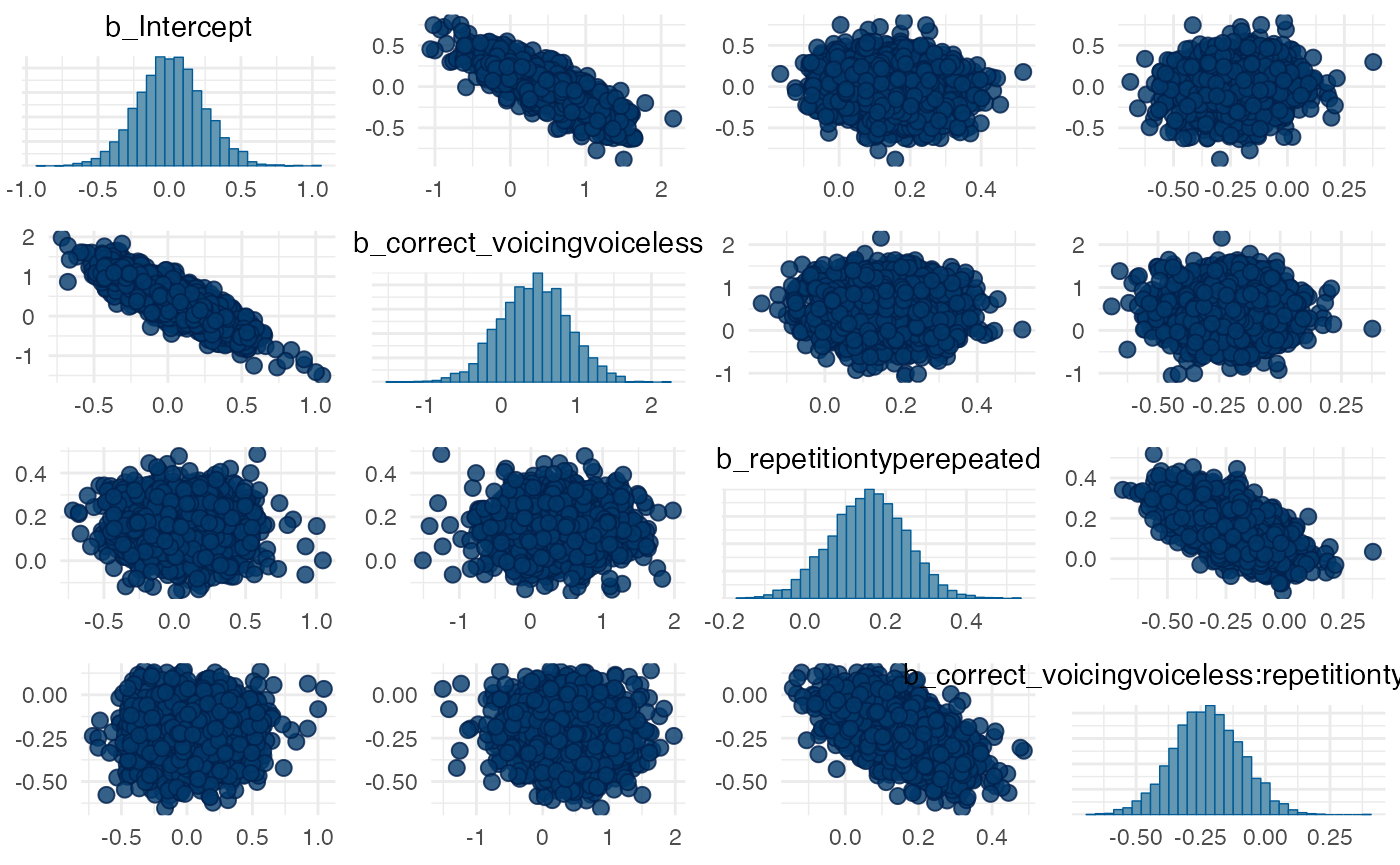# Check Rhat and ESS (remove the rest of the output so it doesn't distract)
summary(m1_full)\$fixed[, 5:7]
#>                                                      Rhat Bulk_ESS Tail_ESS
#> Intercept                                       1.0049467      978     1358
#> correct_voicingvoiceless                        1.0045582     1227     1982
#> repetitiontyperepeated                          1.0013905     3885     2915
#> correct_voicingvoiceless:repetitiontyperepeated 0.9999375     4264     2502

## Prior sensitivity analysis

m1_fixed <- tidy(m1_full, effects = "fixed", conf.level = 0.95, fix.intercept = FALSE) %>%
mutate(
ci_width = abs(conf.low - conf.high)
)
#> Warning in tidy.brmsfit(m1_full, effects = "fixed", conf.level = 0.95,
#> fix.intercept = FALSE): some parameter names contain underscores: term naming
#> may be unreliable!
m1_fixed
#> # A tibble: 4 x 8
#>   effect component term           estimate std.error conf.low conf.high ci_width
#>   <chr>  <chr>     <chr>             <dbl>     <dbl>    <dbl>     <dbl>    <dbl>
#> 1 fixed  cond      Intercept        0.0125    0.231   -0.437     0.477     0.914
#> 2 fixed  cond      correct_voici…   0.439     0.461   -0.454     1.33      1.79
#> 3 fixed  cond      repetitiontyp…   0.156     0.0938  -0.0272    0.336     0.363
#> 4 fixed  cond      correct_voici…  -0.224     0.133   -0.486     0.0319    0.518
m1_fixed %>%
mutate(
theta = c(0, 0, 0, 0),
sigma_prior = c(3, 1, 1, 1),
z = abs((estimate - theta) / std.error), # it's called here std.error but is the standard deviation
s = 1 - (std.error^2 / sigma_prior^2)
) %>%
ggplot(aes(s, z, label = term)) +
geom_point() +
geom_label_repel(arrow = arrow()) +
xlim(0, 1) + ylim(0, 5)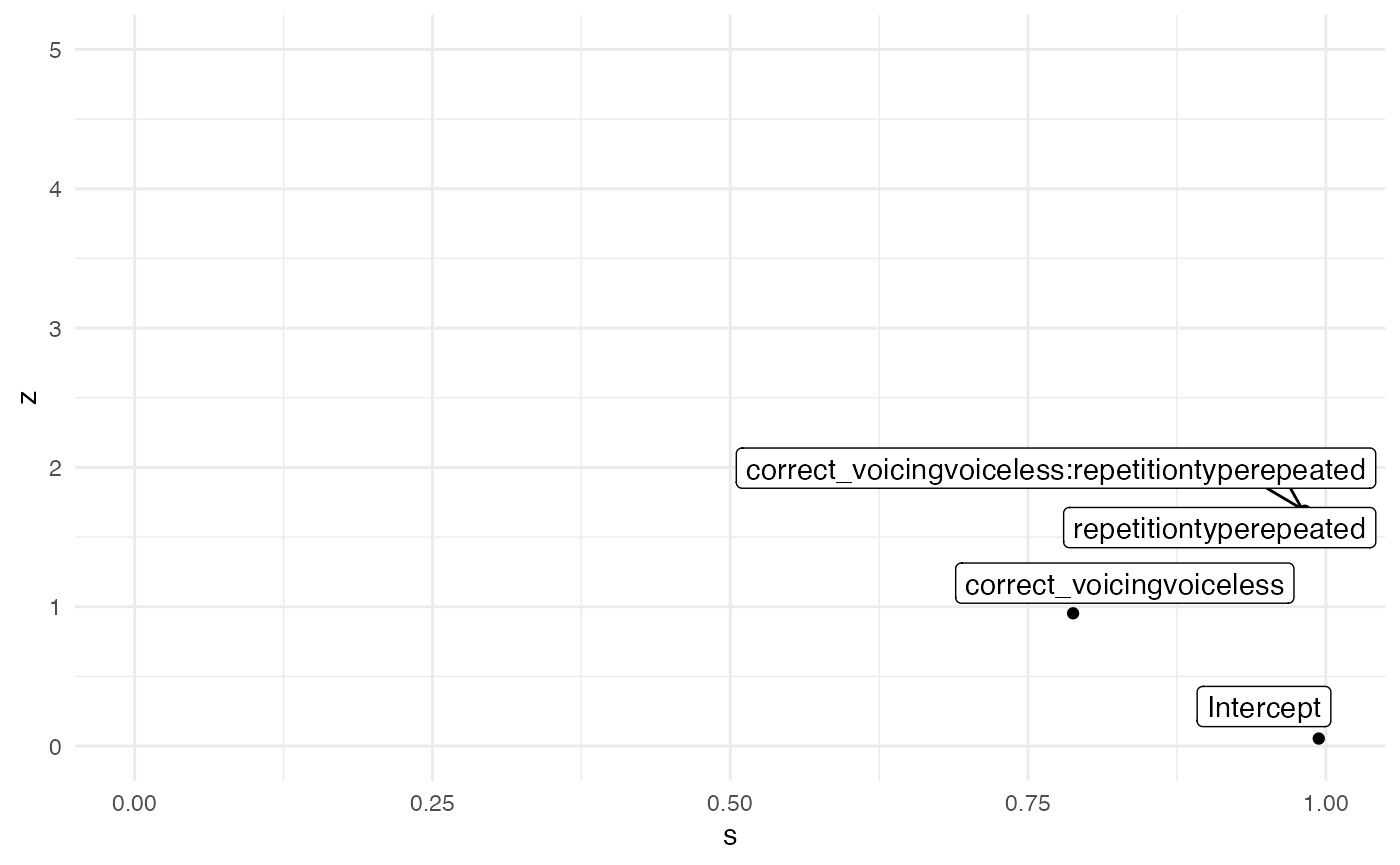labels <- tibble(
x = c(0.25, 0.25, 0.6, 0.75),
y = c(1.25, 3.75, 1.25, 3.75),
labs = c("Poorly identified", "Prior/Posterior\nconflict", "Ideal", "Overfit")
)

m1_fixed %>%
mutate(
theta = c(0, 0, 0, 0),
sigma_prior = c(3, 1, 1, 1),
z = abs((estimate - theta) / std.error), # it's called here std.error but is the standard deviation
s = 1 - (std.error^2 / sigma_prior^2)
) %>%
ggplot(aes(s, z, label = term)) +
annotate("rect", xmin = 0, ymin = 0, xmax = 0.5, ymax = 2.5, alpha = 0.5, fill = "#e66101") +
annotate("rect", xmin = 0, ymin = 2.5, xmax = 0.5, ymax = 5, alpha = 0.5, fill = "#fdb863") +
annotate("rect", xmin = 0.5, ymin = 0, xmax = 1, ymax = 2.5, alpha = 0.5, fill = "#b2abd2") +
annotate("rect", xmin = 0.5, ymin = 2.5, xmax = 1, ymax = 5, alpha = 0.5, fill = "#5e3c99") +
geom_label(data = labels, aes(x, y, label = labs), colour = "white", fill = "black") +
geom_label_repel(fill = NA) +
geom_point() +
xlim(0, 1) + ylim(0, 5)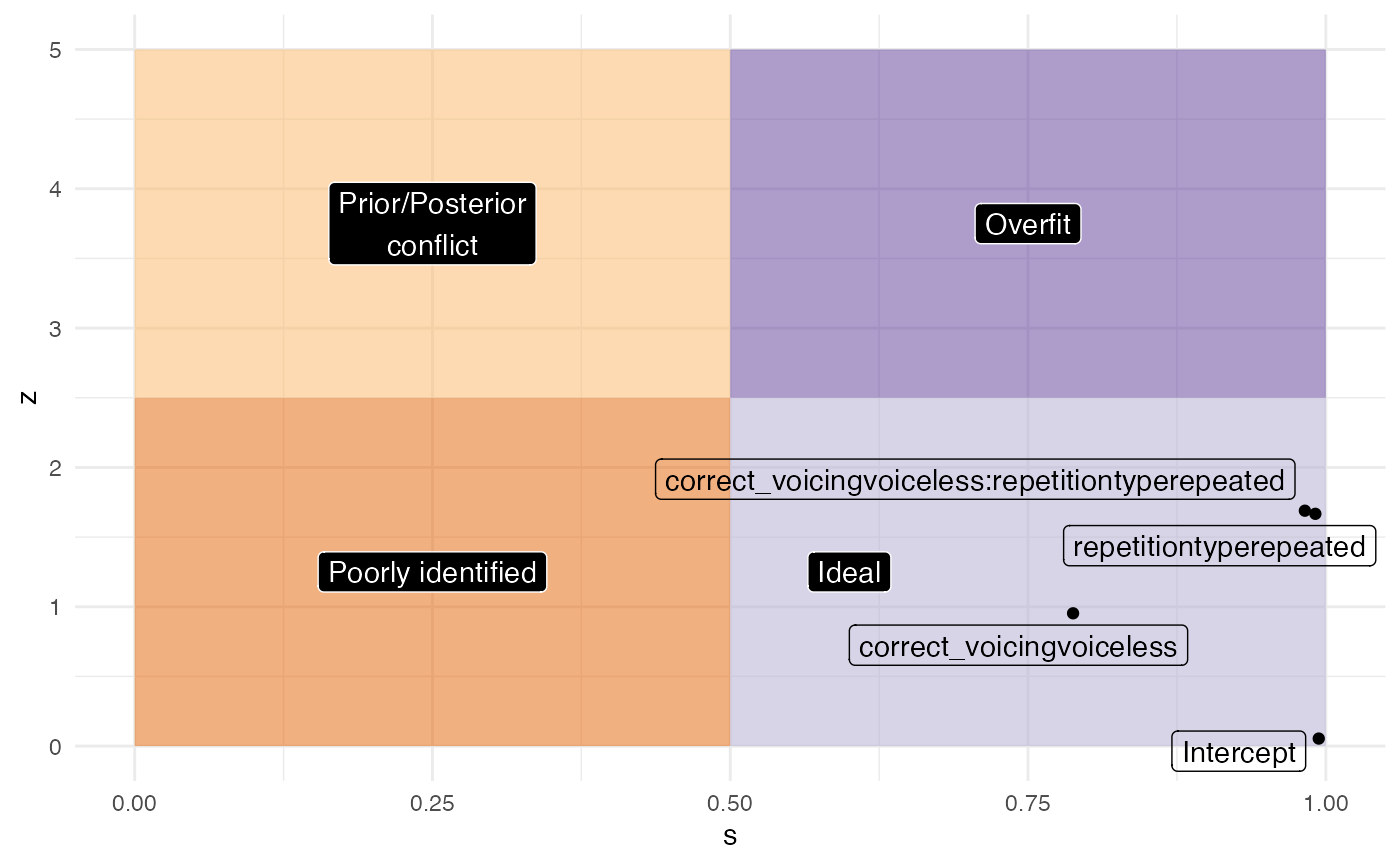# Visual effects

A quick and dirty way to assess the posterior predictions is using the conditional_effects() function. It is also useful because it plots the data into the original scale.

# quick and dirty plot on the original scale
plot(conditional_effects(m1_full), ask = FALSE)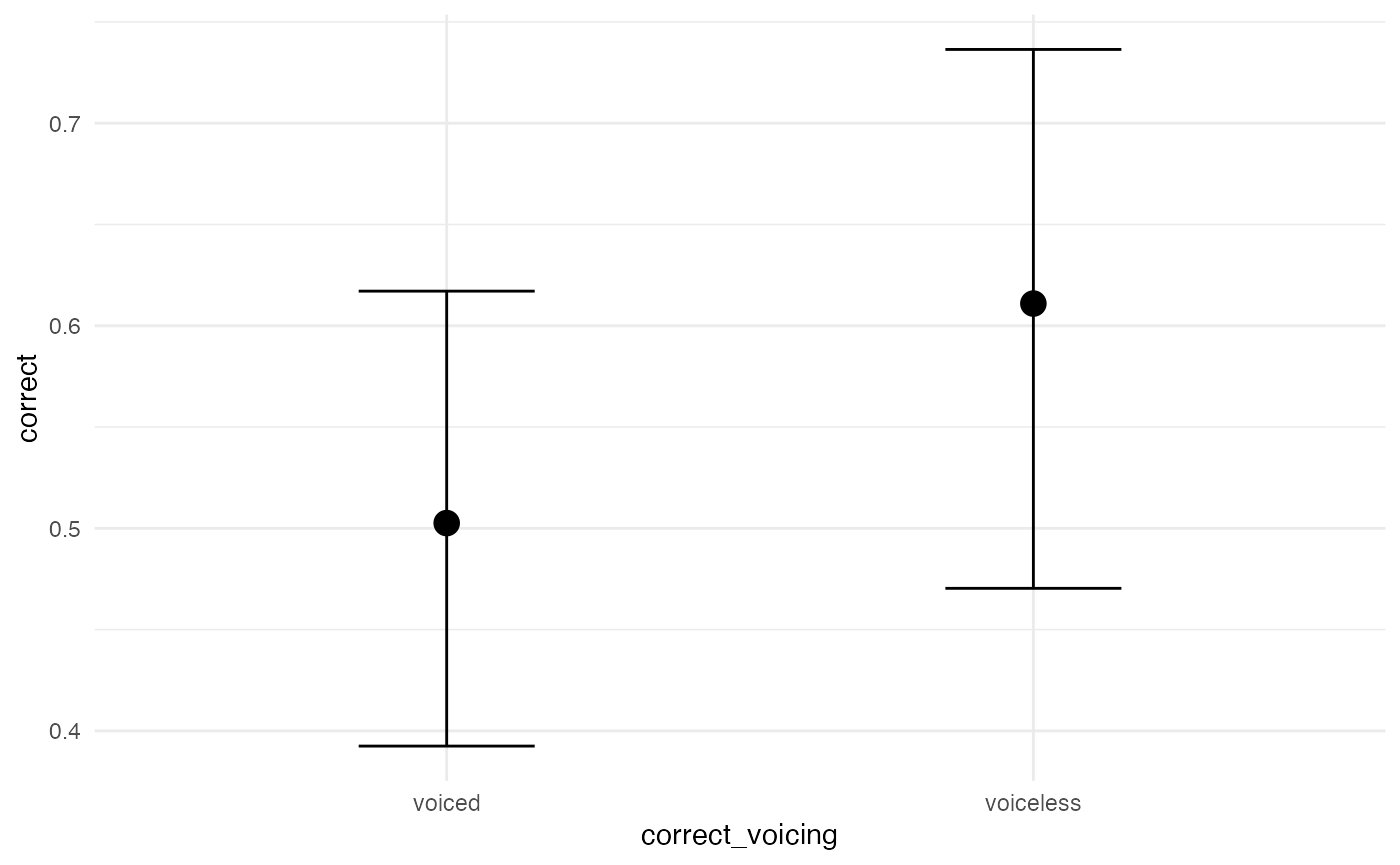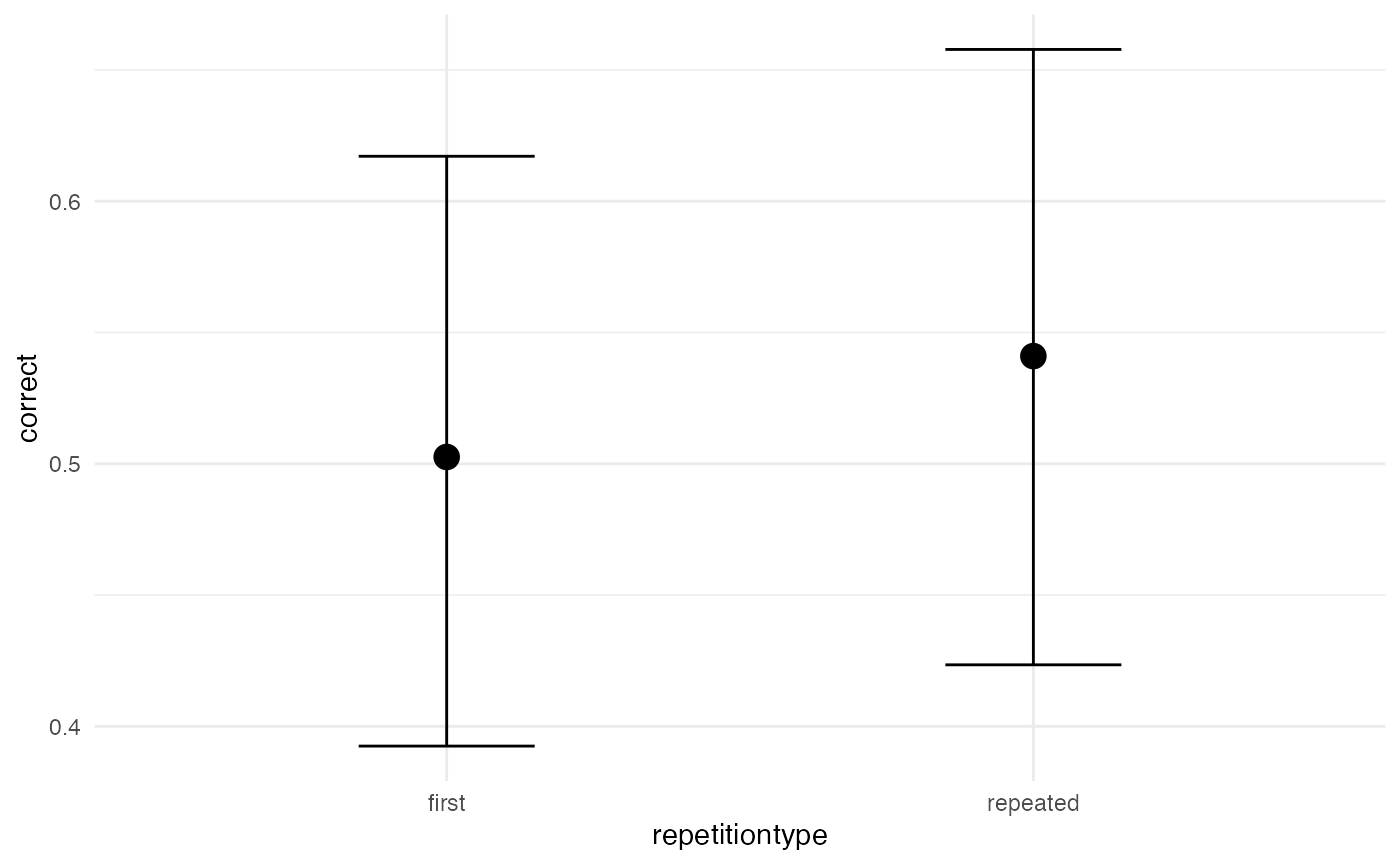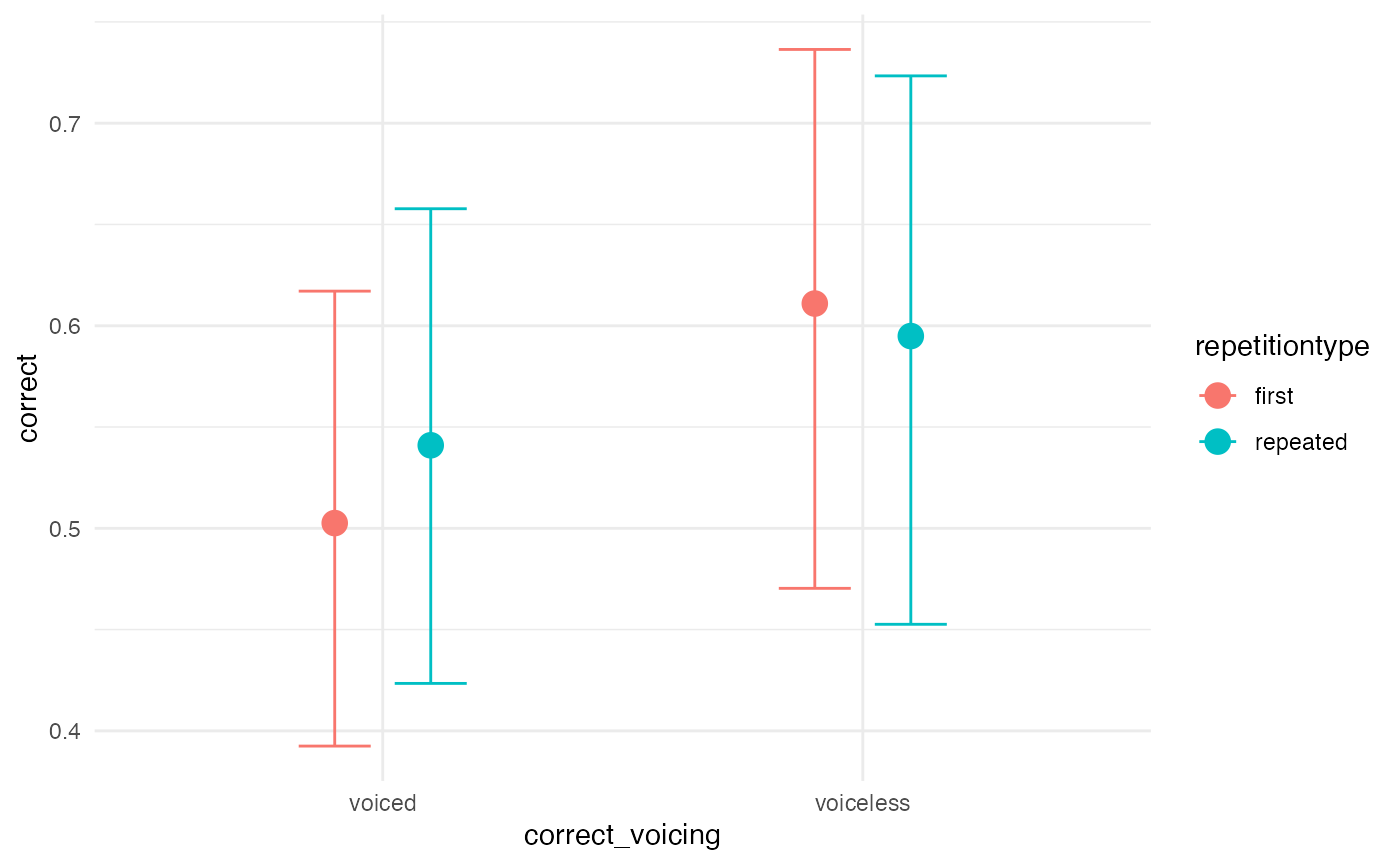We can also plot the posterior distributions of our population-level coefficients. This can be conveniently done with the bayesplot package.

posterior <- as.matrix(m1_full)

mcmc_areas(posterior,
pars = c("b_Intercept",
"b_correct_voicingvoiceless",
"b_repetitiontyperepeated",
"b_correct_voicingvoiceless:repetitiontyperepeated"),
# arbitrary threshold for shading probability mass
prob = 0.83)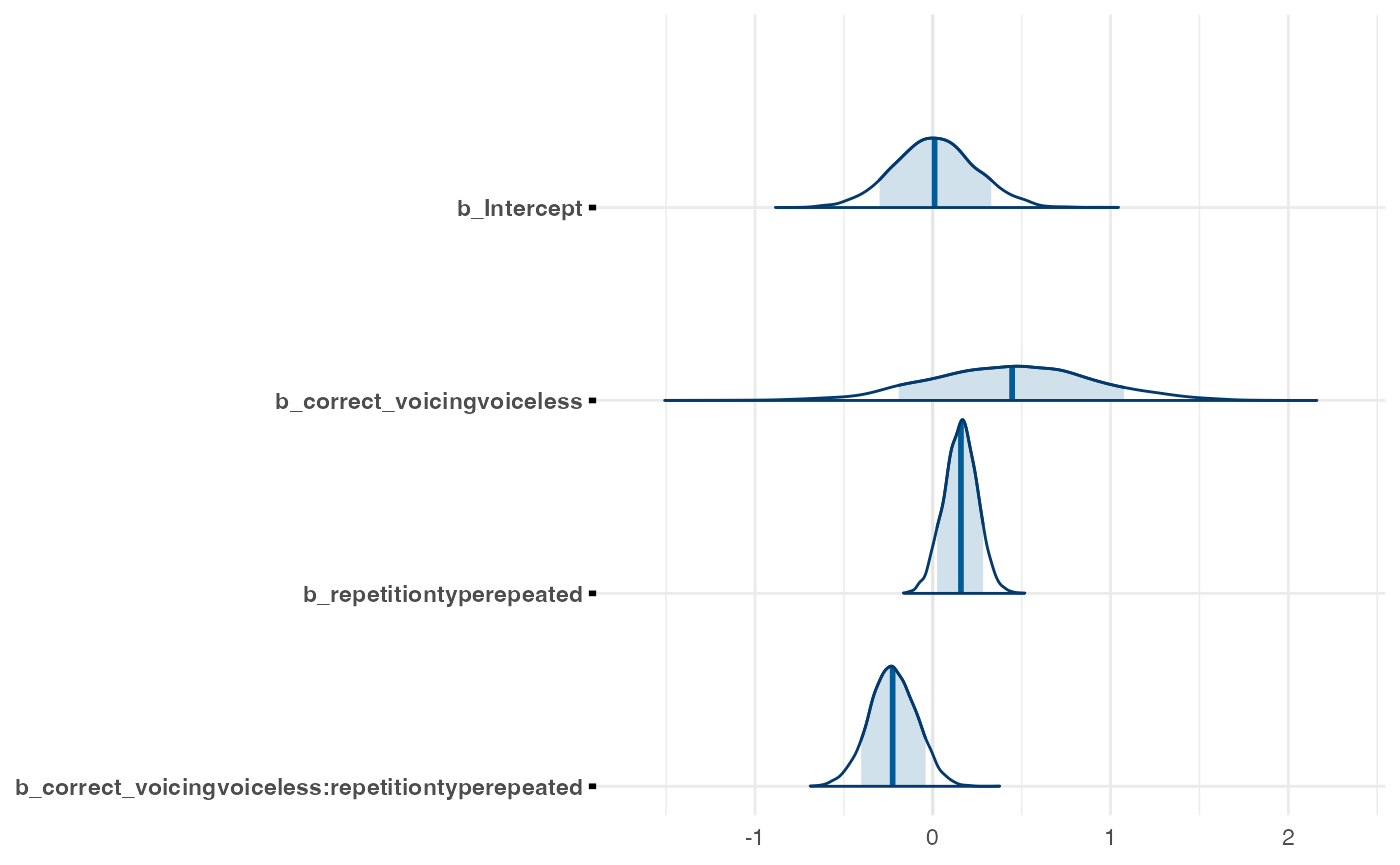For more traditional plotting of the actual levels of the predictors, we can use the data_grid() and add_fitted_draws() functions. Here is an example.

post_data <- incomplete %>%
data_grid(correct_voicing, repetitiontype) %>%
add_fitted_draws(m1_full, n = 4000, re_formula = NA)

post_plot <- post_data %>%
# plot
ggplot(aes(y = .value, x = correct_voicing,
fill = correct_voicing)) +
# density plus CrIs
stat_halfeye() +
# reference line at chance level = 0.5
geom_hline(yintercept = 0.5, lty = "dashed") +
# split by repetition type
facet_grid(~repetitiontype) +
# color code
scale_fill_manual(values = c("#8970FF", "#FFA70B")) +
#scale_color_manual(values = c("#8970FF", "#FFA70B")) +
# rename y axis
labs(y = "probability of correct",
x = "underlying voicing")
post_plot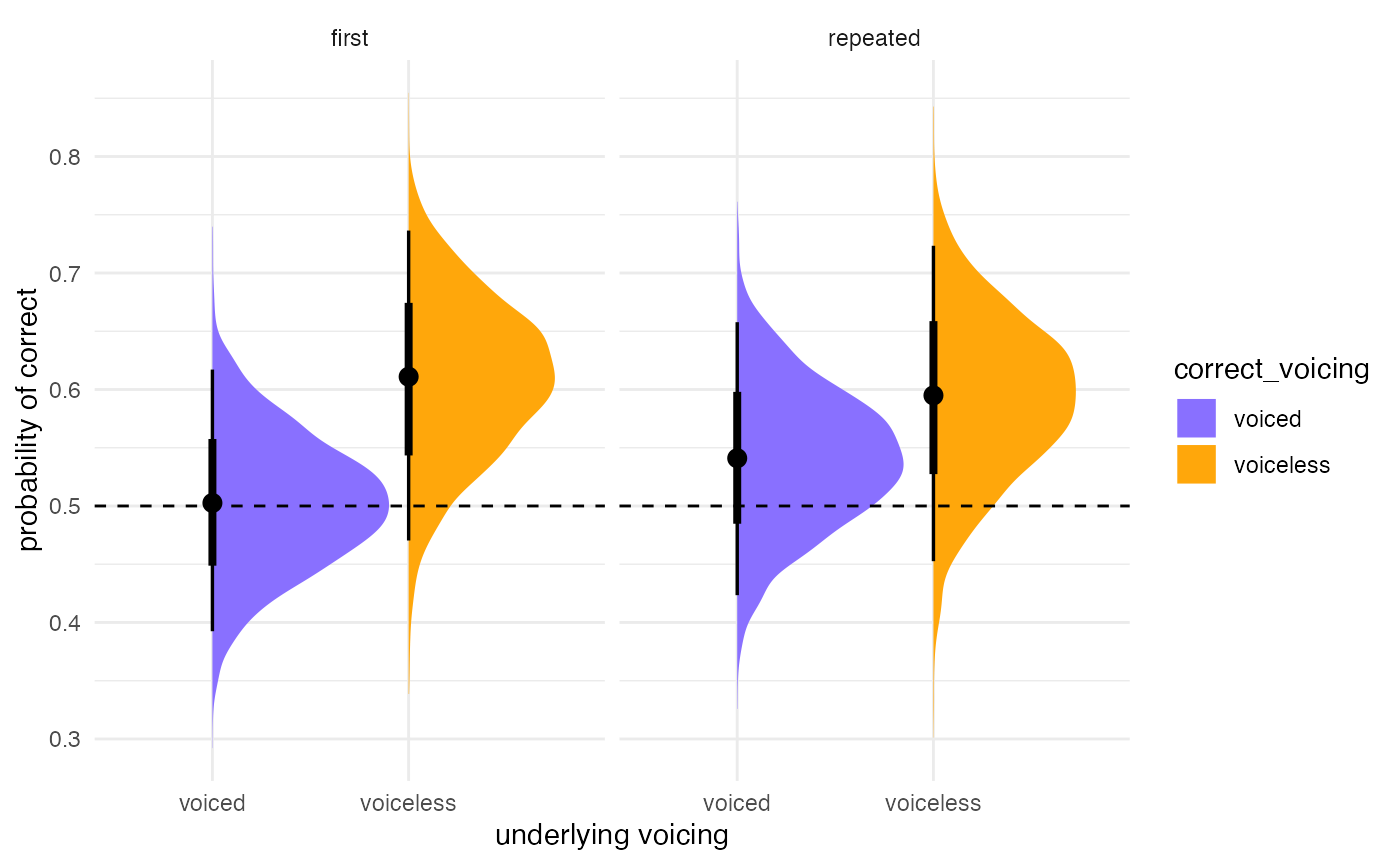If you want to add the actual aggregated accuracy for each listener to the plot (for example), you can add that information on top. Makes for a very informative plot.

#aggregate
incomplete_agg <- incomplete %>%
group_by(listener, correct_voicing, repetitiontype) %>%
summarise(.value = mean(as.numeric(correct)), .groups = "drop")

post_plot +
geom_point(data = incomplete_agg,
aes(y = .value,
x = correct_voicing,
fill = correct_voicing),
pch = 21, alpha = 0.5,
position = position_nudge(x = -0.1))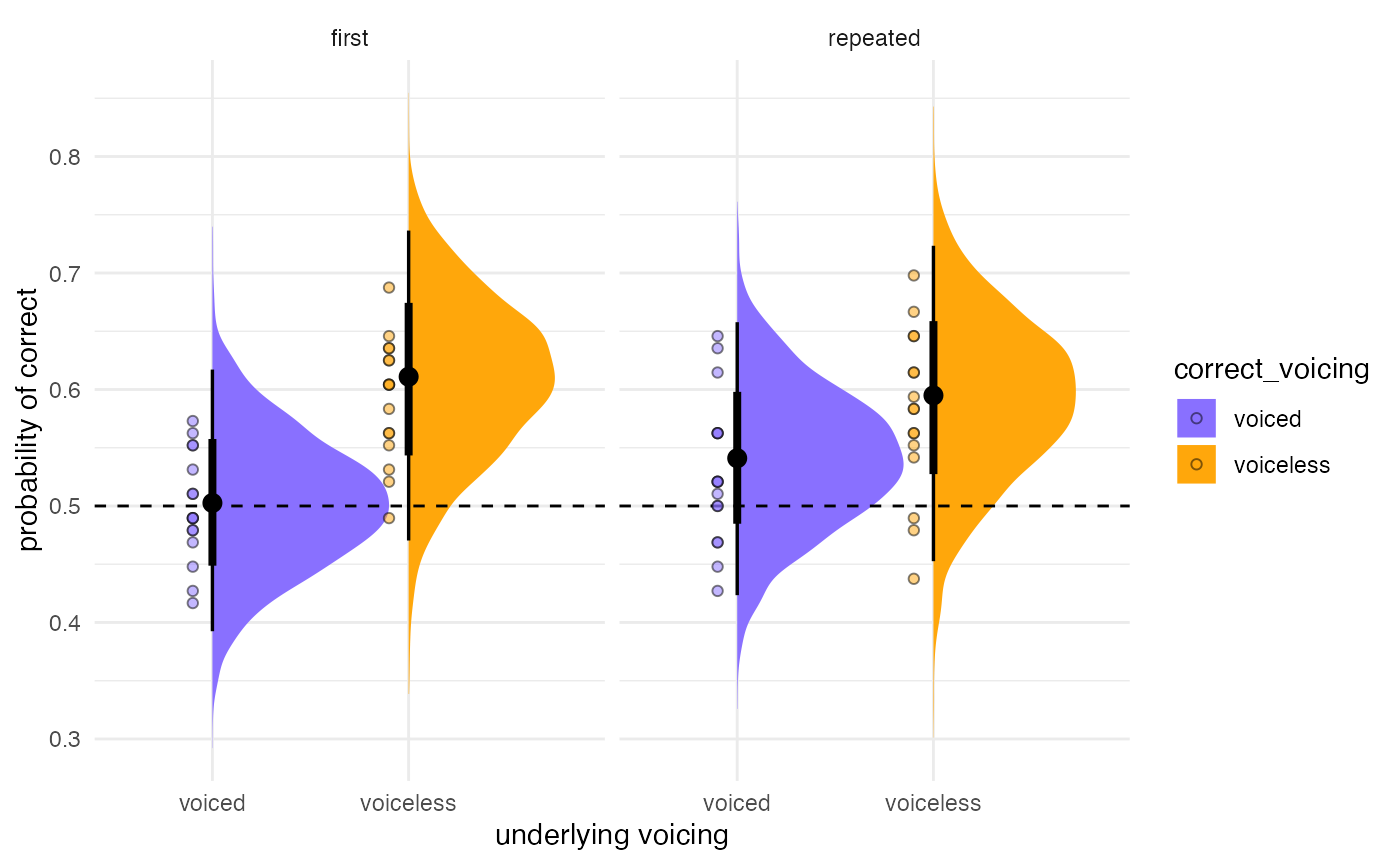# Inference

## Interpreting model output

# Describe posterior using fixef (to get simple, clean output...
# focus on understanding each estimate in context and examining CrI's)
# Use in conjuction with levels_plot-2
fixef(m1_full)
#>                                                    Estimate  Est.Error
#> Intercept                                        0.01248959 0.23130872
#> correct_voicingvoiceless                         0.43899959 0.46065151
#> repetitiontyperepeated                           0.15628813 0.09375277
#> correct_voicingvoiceless:repetitiontyperepeated -0.22414533 0.13267918
#>                                                        Q2.5      Q97.5
#> Intercept                                       -0.43687340 0.47723123
#> correct_voicingvoiceless                        -0.45357875 1.33148511
#> repetitiontyperepeated                          -0.02722672 0.33584627
#> correct_voicingvoiceless:repetitiontyperepeated -0.48584540 0.03186847
# Focus on describing posterior
hdi_range <- bayestestR::hdi(m1_full, ci = c(0.65, 0.70, 0.80, 0.89, 0.95))
hdi_range
#> Highest Density Interval
#>
#> Parameter                                       |        65% HDI |        70% HDI |        80% HDI |        89% HDI |       95% HDI
#> -----------------------------------------------------------------------------------------------------------------------------------
#> (Intercept)                                     | [-0.21,  0.21] | [-0.25,  0.23] | [-0.27,  0.32] | [-0.36,  0.37] | [-0.46, 0.45]
#> correct_voicingvoiceless                        | [ 0.01,  0.87] | [-0.01,  0.93] | [-0.16,  1.02] | [-0.29,  1.16] | [-0.47, 1.31]
#> repetitiontyperepeated                          | [ 0.07,  0.25] | [ 0.07,  0.26] | [ 0.03,  0.27] | [ 0.00,  0.30] | [-0.03, 0.34]
#> correct_voicingvoiceless:repetitiontyperepeated | [-0.35, -0.11] | [-0.36, -0.09] | [-0.39, -0.05] | [-0.42,  0.00] | [-0.49, 0.03]

plot(hdi_range, show_intercept = T)